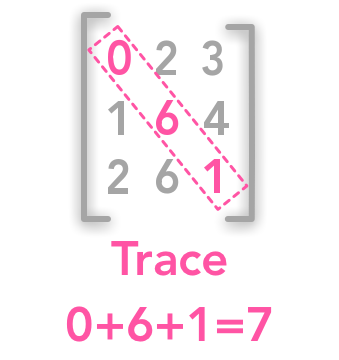# Introduction

I can assure you that you will read this chapter in 2 minutes! It is nice after the last two chapters that were quite big! We will see what is the Trace of a matrix. It will be needed for the last chapter on the Principal Component Analysis (PCA).

# 2.10 The Trace OperatorThe trace of matrix

The trace is the sum of all values in the diagonal of a square matrix.

$\bs{A}= \begin{bmatrix} 2 & 9 & 8 \\ 4 & 7 & 1 \\ 8 & 2 & 5 \end{bmatrix}$

$\mathrm{Tr}(\bs{A}) = 2 + 7 + 5 = 14$

Numpy provides the function trace() to calculate it:

A = np.array([[2, 9, 8], [4, 7, 1], [8, 2, 5]])
A

array([[2, 9, 8],
[4, 7, 1],
[8, 2, 5]])

A_tr = np.trace(A)
A_tr

14


GoodFellow et al. explain that the trace can be used to specify the Frobenius norm of a matrix (see 2.5). The Frobenius norm is the equivalent of the $L^2$ norm for matrices. It is defined by:

$\norm{\bs{A}}_F=\sqrt{\sum_{i,j}A^2_{i,j}}$

Take the square of all elements and sum them. Take the square root of the result. This norm can also be calculated with:

$\norm{\bs{A}}_F=\sqrt{\Tr({\bs{AA}^T})}$

We can check this. The first way to compute the norm can be done with the simple command np.linalg.norm():

np.linalg.norm(A)

17.549928774784245


The Frobenius norm of $\bs{A}$ is 17.549928774784245.

With the trace the result is identical:

np.sqrt(np.trace(A.dot(A.T)))

17.549928774784245


Since the transposition of a matrix doesn’t change the diagonal, the trace of the matrix is equal to the trace of its transpose: $\Tr(\bs{A})=\Tr(\bs{A}^T)$

## Trace of a product

$\Tr(\bs{ABC}) = \Tr(\bs{CAB}) = \Tr(\bs{BCA})$

### Example 1.

Let’s see an example of this property.

$\bs{A}= \begin{bmatrix} 4 & 12 \\ 7 & 6 \end{bmatrix}$

$\bs{B}= \begin{bmatrix} 1 & -3 \\ 4 & 3 \end{bmatrix}$

$\bs{C}= \begin{bmatrix} 6 & 6 \\ 2 & 5 \end{bmatrix}$

A = np.array([[4, 12], [7, 6]])
B = np.array([[1, -3], [4, 3]])
C = np.array([[6, 6], [2, 5]])

np.trace(A.dot(B).dot(C))

531

np.trace(C.dot(A).dot(B))

531

np.trace(B.dot(C).dot(A))

531


$\bs{ABC}= \begin{bmatrix} 360 & 432 \\ 180 & 171 \end{bmatrix}$

$\bs{CAB}= \begin{bmatrix} 498 & 126 \\ 259 & 33 \end{bmatrix}$

$\bs{BCA}= \begin{bmatrix} -63 & -54 \\ 393 & 594 \end{bmatrix}$

$\Tr(\bs{ABC}) = \Tr(\bs{CAB}) = \Tr(\bs{BCA}) = 531$

Feel free to drop me an email or a comment. The syllabus of this series can be found in the introduction post. All the notebooks can be found on Github.

This content is part of a series following the chapter 2 on linear algebra from the Deep Learning Book by Goodfellow, I., Bengio, Y., and Courville, A. (2016). It aims to provide intuitions/drawings/python code on mathematical theories and is constructed as my understanding of these concepts. You can check the syllabus in the introduction post.